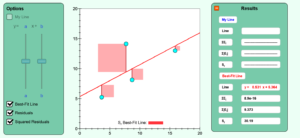# Chapter 6 Guess the Linear Regression Model Simulation

Description:  This simulation depicts linear regression.Keywords:  regression, linear regression, sum of square of residuals

Learning Objectives:  After successful completion of running the simulation with proper questions asked by the instructor, the student would be able to 1) note geometrically what the sum of the squared residuals mean 2) see how close they come to guessing the linear regression curve.

Full Resources:  The full resources for the topic of the linear regression models are given here which include textbook content, a PowerPoint presentation, a multiple-choice test, audiovisual lectures, and application examples.